## Example Questions

← Previous 1

### Example Question #1 : How To Find The Amount Of Sales Tax

If there is a 10% sale on an item, and then 9% sales tax is applied to that after-sale price, then what is the total cost of the item including tax as a percentage of its pre-sale sticker price?

98.1%

101%

81.9%

99%

99.2%

98.1%

Explanation:

A 10% sale means that the post-sale price of the item is now 90%, or 0.9 of the original cost of the item. We then apply 9% sales tax by multiplying the 0.9 by 109%, or 1.09. 0.9 * 1.09 = .981, so the total cost of the item is 98.1% of the original pre-sale sticker price.

For percentage problems that do not deal with a specific starting number, it is always helpful to plug in 100 for the starting number. Here, we would then have a post-sale price of 90 dollars, and if we calculate the sales tax for the 90-dollar item it would be 90 * 0.09 = $8.10. THis gives us a total cost of 90 + 8.10 =$98.10, or 98.1% of the original 100-dollar price.

### Example Question #2 : How To Find The Amount Of Sales Tax

Cynthia buys 2 shirts, each costing $35, a pair of pants for$40 and a belt for $18. At the register the total she owes is$138.24.  What is the rate of sales tax in her state?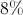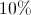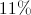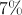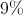Explanation:

To find the amount of sales tax, take the difference in the total before and after tax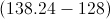and divide by the price before tax.  This gives 0.08 or 8%.

A certain state charges sales tax at a rate of 8.75% per dollar spent. John spends $127.50 total, including tax, on clothes. What was the total price of the clothing before tax was added? Possible Answers: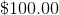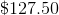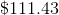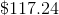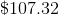Correct answer:Explanation: Since we know the total price with tax, we simply need to work backwards to remove the tax from the total price and find the price of the clothing itself. This can be done as follows: Clothing Price or C = Total price / 1 + tax rate C =$127.50/(1 + 0.0875) = $117.24 ### Example Question #1 : How To Find The Amount Of Sales Tax Jack is at the grocery store. He buys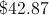in groceries, and groceries are not taxed in his state. He also buys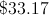in other items, which are taxed at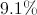(not yet applied). How much is his total? Possible Answers: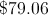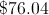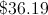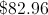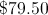Correct answer:Explanation: Jack's groceries are not taxed, so they cost, but his other items are taxed. We will calculate the price with the tax: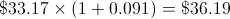We will add the two together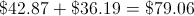### Example Question #5 : How To Find The Amount Of Sales Tax Harry, on his first lunch date with Sally, decides to put the entire bill on his credit card. If the bill came to$28 and Harry wants to leave an 18% tip, what is the total amount that he should pay?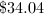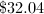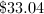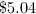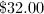Explanation:

To find an 18% increase, multiply by 1.18.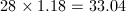### Example Question #121 : Percentage

A dealership is selling a used car with a sticker price of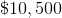. If state sales tax is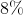, how much will the taxes on purchasing the car be?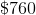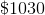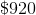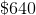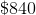Explanation:

The amount paid in sales tax is determined by multiplying the decimal value of the tax rate by the sticker price of the car: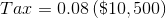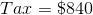### Example Question #1 : How To Find The Amount Of Sales Tax

Jenny buys a blouse that is priced at $45. She pays a total of$48.15, what is the rate of tax on the blouse?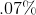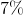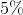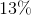Explanation:

The purpose of this question is to calculate tax rates using dollar amounts.

First, the amount of tax payed must be determined. This is done by finding the difference between the amount paid and the listed price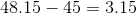,

which equals $3.15. Then, that must be translated into a percentage of$45.

Therefore,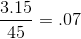, yielding .07 of 1. This is a 7% tax rate.

### Example Question #10 : How To Find The Amount Of Sales Tax

Billy is buying from a sudden inflated economy.  Suppose a sales tag shows the price of a shirt to be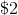.  Right at checkout, the cashier charged Billy a hefty sales tax that made the shirt cost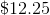.  What percent was this sales tax?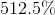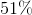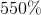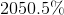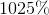Explanation:

In order to answer this question, we need to set up an equation such that we are adding the cost of the shirt with some percentage of the shirt's cost that will equal the final price.

A percentage is some value out of 100 parts.

Write the equation.  We will solve for, which represents the percentage.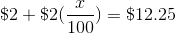Subtract 2 from both sides and eliminate the dollar signs.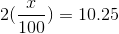Reduce the left hand side of the equation.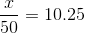Multiply by 50 on both sides.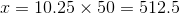This number is the percentage sales tax that was applied to the shirt.

The answer is:← Previous 1

### All SAT Math Resources